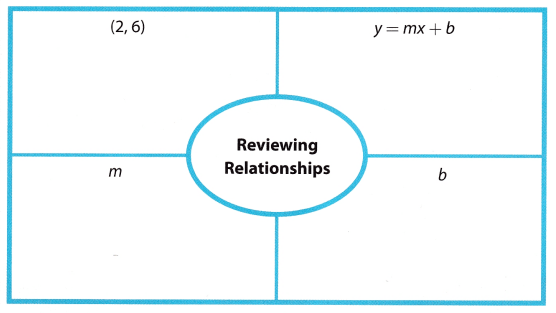Refer to our Texas Go Math Grade 8 Answer Key Pdf to score good marks in the exams. Test yourself by practicing the problems from Texas Go Math Grade 8 Module 6 Answer Key Functions.

Complete these exercises to review skills you will need for this chapter.

Evaluate each expression for the given value of x.

Question 1.
2x + 3 for x = 3 ____
2x + 3 for x = 3 Given
2(3) + 3 Substitute the given value of x for x
6 + 3 Multiply

Question 2.
-4x + 7 for x = -1 _____
To evaluate an expression for the given value of x, let us substitute the value of x in the expression.

Evaluating -4x + 7 for x = -1
-4x + 7 = -4(-1) + 7 Substitute the value of x.
= 4 + 7 Simplify.
= 11

Question 3.
1.5x – 2.5 for x = 3 _____
1.5x – 2.5 for x = 3 Given
1.5(3) – 2.5 Substitute the given value of x for x
4.5 – 2.5 Multiply
2 Subtract

Question 4.
0.4x + 6.1 for x = -5 ______
0.4x + 6.1 for x = -5 Given
0.4(-5) + 6.1 Substitute the given value of x for x
-2 + 6.1 Multiply

Question 5.
$$\frac{2}{3}$$x – 12 for x = 18 _____
$$\frac{2}{3}$$x – 12 for x = 18 Given
$$\frac{2}{3}$$(18) – 12 Substitute the given value of x for x
12 – 12 Multiply
0 subtract

Question 6.
–$$\frac{5}{8}$$x + 10 for x = -8 ______
To evaluate an expression for the given value of x, let us substitute the value of x in the expression.

Evaluating –$$\frac{5}{8}$$x + 10 for x = -8
–$$\frac{5}{8}$$x + 10 = –$$\frac{5}{8}$$(-8) + 10 Substitute the value of x.
= $$\frac{40}{8}$$ + 10 Simplify.
= 5 + 10
= 15

Define the variables for each situation. Then write an equation.

Question 7.
Jana’s age plus 5 equals her sister’s age.
j = Janas age Define the variables used in the situation
s = Jana’s sister age

s = j + 5 Identify the operation involved. Plus indicated addition

Question 8.
Andrew’s class has 3 more students than Lauren’s class.
A = Number in Andrew’s class Define the variables used in the situation
L = Number in Lauren’s class

A = L + 3 Identify the operation involved. more indicated addition

Question 9.
The bank is 50 feet shorter than the firehouse.
Given the statement The bank is 50 feet shorter than the firehouse’ the variables can be defined by letting x be the height of the bank; and y be the height of the firehouse.

Since x represents the height of the bank that is 50 feet shorter that the firehouse represented as variable y, we
can equate x to y – 50 that gives an equation
x = y – 50

Question 10.
The pencils were divided into 6 groups of 2.
g = number in pencils in groups Define the variables used in the situation
n = number of groups

$$\frac{12}{n}$$ = g Identify the operation involved. divided indicated division

Visualize Vocabulary
Use the ✓ words to complete the diagram. You can put more than one word in each section of the diagram.Understand Vocabulary

Complete the sentences using the preview words.

Question 1.
A rule that assigns exactly one output to each input is a ___.
A rule that assigns exactly one output to each input is a function.

Explanation:
A function is defined as the relationship in which the input value is paired with exactly one output value.
By definition, a rule that assigns exactly one output to each input is a function.
Thus, the blank part of the statement is function

Question 2.
The value that is put into a function is the ___________
The value that is put into a function is the input

Explanation:
In a function, we have two types of values the input and the output values. Input value is defined as the independent variable that is being put into a function. It affects the output because output is the dependent variable or the result in a function.

By definition, the value that is put into a function is input

Thus, the blank part of the statement is input.

Question 3.
The result after applying the function machine’s rule is the ____.